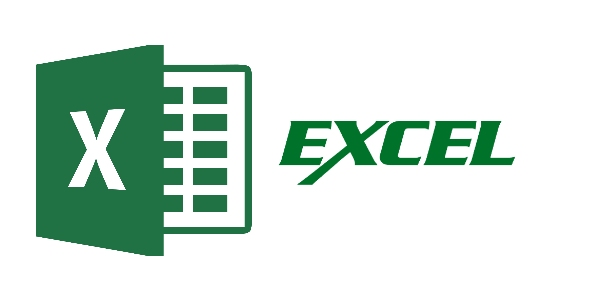# Excel Test Spread Sheeting And Graphing

16 Questions | Total Attempts: 242Settings• 1.
Explain the difference between AND and OR statements.
• 2.
Explain what recursively means?  Give an example relating to Excel.
• 3.
Why will the following formula not work.   =IF(A1>A2,”true”,Not true) when A1 is equal to 25 000 and A2 is equal to 23000
• 4.
When calculating cells what does a colon allow you to do?
• A.

Find the mean median and mode of a series of numbers

• B.

Calculate multiple cells by typing them in

• C.

Calculate multiple cells by highlighting them

• D.

Produce conditional statements like if and or not

• 5.
What is the correct answer given the formula ROUNDUP (A4,3), when A4 is equal to 12.34912?
• A.

12.349

• B.

12.35

• C.

12

• D.

12.348

• 6.
What is the correct answer given the formula SMALL(B2-H1,3), for the following table A B C D E F G H I J 1 4 3 6 9 12 2 11 5 3 3 6 10 5 7 9 14 2 1 7
• A.

1

• B.

2

• C.

3

• D.

4

• 7.
What is the correct answer given the formula MEDIAN(A2-A7) for the following  table.   1 2 3 4 5 6 7 8 9 A 2 5 7 9 13 23 4 16 1
• A.

9

• B.

10.5

• C.

9.5

• D.

11.5

• 8.
All formulas in Excel must be begin with what symbol
• A.

=

• B.

SUM

• C.

,

• D.

(

• 9.
What is the correct formula to solve for the Interest of following graph? A B C D E Year Opening Balance(\$) Interest Rate (%) Annual investment(\$) Closing Balance (\$) 1 1000.00 6 1000.00 2060
• A.

=B3*0.06+D3

• B.

=B3*0.06

• C.

=B3*6+D3

• D.

=B3*6

• 10.
The graphing functions in Microsoft Excel can be found in what tab.
• A.

Graphing

• B.

Insert

• C.

Formulas

• D.

Home

• 11.
When is the best situation to use a pie graph?
• A.

When observing trends in a population

• B.

When observing changes over time

• C.

When comparing one category to the group

• D.

When comparing one category to another

• 12.
The "Formula" in excel is...
• A.

A tab you see at the bottom of your workbook file.

• B.

A combination of numbers and symbols used to express a calculation.

• C.

To enter text or numbers at the position of the cursor or cell pointer.

• D.

The lines on your worksheet that separate the columns and rows.

• E.

A place in a Microsfoft Excel window where you type information, such as a file name.

• 13.
What is Microsoft Excel?
• A.

A program that creates and edits newsletters, brochures, flyers, and web sites.

• B.

A program that creates spreadsheets that combines worksheets, graphics, and data management into a single program.

• C.

A program that creates databases and programs to track and manage information.

• D.

A program designed to create notes and presentations.

• E.

A word processing program designed to create letters, documents, or reports.

• 14.
What answer will be provided given the formula =IF(A1>B2) for the cell values.   A B 1 5 6 2 3 4
• A.

Error

• B.

Number

• C.

Value

• D.

False

• E.

True

• 15.
Based on the graph #1 in the assignments and hand out section of our website, what two years did the CIS boys volleyball team achieve the same amount of wins?
• A.

2010 and 2012

• B.

2009 and 2010

• C.

2010 and 2011

• D.

2011 and 2012

• 16.
Based on the graph #1 in the assignments and hand out section of our website, in what year did the CIS girl’s volleyball achieve more wins than the boys?
• A.

2011

• B.

2009

• C.

2013

• D.

2012

Related TopicsBack to top